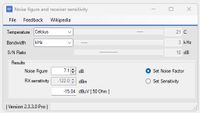# Noise and receiver sensitivity calculator.To be introduced in V2330

Use this calculator to calculate Receiver sensitivity ( Signal level to obtain a specified number of dB's Signal-to-Noise ratio SNR ) Set temperature in Fahrenheit, Celsius or Kelvin. Specify the bandwidth and SNR and Noise Factor ( Set noise factor) to obtain the sensitivity in dBm or dBuV (50 Ohms).

In case the Set Sensitivity is selected, the required Noise Factor can be calculated for the system.

>100 RF Calculators
WinRFCalc, the best RF calculator toolbox for Windows